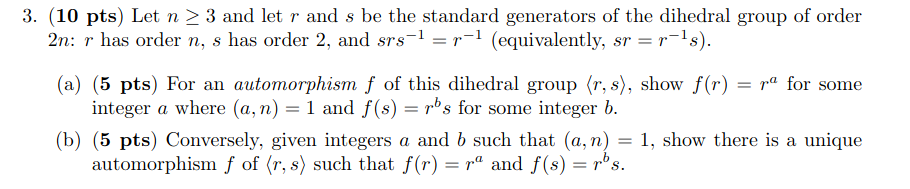## Generators

A group is finitely generated if there is a finite set $A$ which generates it.

• finite groups are finitely generated
• $\Z$ is finitely generated
• $\Q$ is not finitely generated.

## Special subgroups

If $H$ is normal in $G$ and $G$ is normal in $K$, is $H$ normal in $K$?

If $H$ is normal in $G$, and $f:G\to K$ is a homomorphism, is $f(H)$ normal in $K$? Show $f(G)$ is contained in $N_{K}(f(H))$.

Let $G$ be the dihedral group $D_{2n}$ of symmetries of a regular $n$-gon.

• Show that the subgroup $R$ of rotations is normal.
• Let $H$ be the subgroup generated by a reflection. What is $N_{G}(H)$? What is $C_{G}(H)$?
• What is the center of $G$?
• Draw the lattice of subgroups of $D_{8}$. (See DF, p. 69)

Consider this prelim problem from the August, 2022 prelim.Let $Q$ be the quaternion group with $8$ elements. Draw its lattice of subgroups. (The quaternion group has 8 elements $\lbrace \pm 1, \pm i, \pm j, \pm k\rbrace$ where each of $i,j,k$ satisfy $x^2=-1$ and $ijk=-1$. Compare $Q$ with $D_{8}$.

Show that $Q$ is isomorphic to the subgroup of $\mathrm{SL}_{2}(\Zn{3})$ generated by the matrices $\begin{pmatrix} 1 & 1\cr 1& -1\end{pmatrix}$ and $\begin{pmatrix} 0 & -1 \cr 1 & 0\end{pmatrix}$.

Show that the center of $S_{n}$ is trivial if $n\ge 3$.

What is the normalizer of the rotation group in $\GL_{2}(\R)$? What is its centralizer? Interpret this in terms of group actions.

The affine group $\mathrm{Aff}(\R^{2})$ of the plane is the subgroup of $\GL_{3}(\R)$ consisting of matrices of the form $$\left( \begin{matrix} a & b & x \cr c & d & y \cr 0 & 0 & 1\cr \end{matrix}\right).$$ It acts on points $(u,v)$ viewed as column vectors $$\left[\begin{matrix} u \cr v \cr 1\cr \end{matrix}\right]$$ by matrix multiplication.

Show that $\GL_{2}(\R)$ viewed as the upper triangular block is a normal subgroup of the affine group.

The additive group $\R^{2}$ is a subgroup of the affine group; what is its normalizer?

The subgroup of the affine group where the upper triangular matrix is a rotation matrix (an “orthogonal matrix”) is called the Euclidean group.

### Every group is a permutation group

Theorem (Cauchy): Any group is a subgroup of a permutation group.

Proof: Given $g\in G$, let $f_{g}:G\to G$ be the map $f_{g}(h)=gh$. This gives a map from $G$ to $S(G)$. We have $f_{e}(h)=eh=h$ so $f_{e}$ is the identity map. Also

$f_{ab}(h)=abh=(f_{a}\circ f_{b})(h)$

for any $h\in G$. Since composition of functions is the group operation in $S(G)$, the map $g\mapsto f_{g}$ is a homomorphism from $G\to S(G)$.

Finally, the map $f_{h}$ is the identity map only when $h=e$. So this map is injective.

Make this explicit for $S_{3}$.